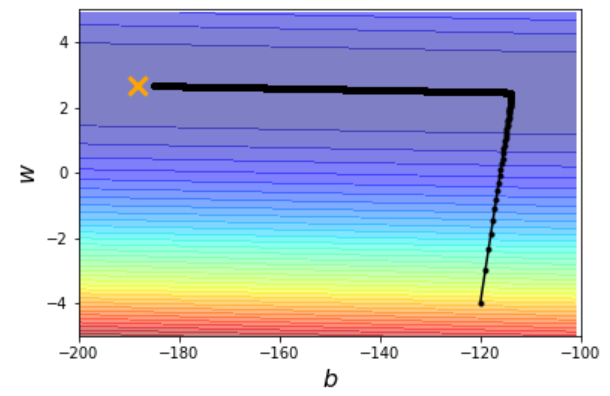# 回归(Regression)

## KeyWords

• Linear Model（线性模型）
• Loss Function（损失函数）
• Generalization（泛化）
• Overfitting（过拟合）
• Regularization（正则化）

## Regression可以做什么

• 股票市场的预测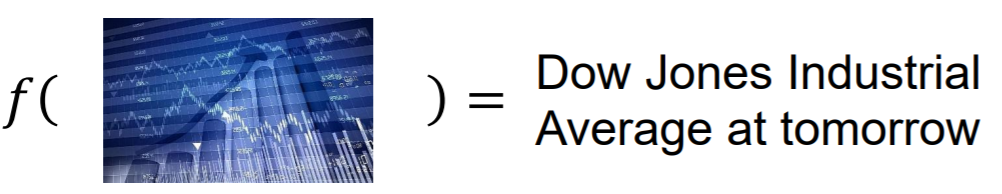• 无人驾驶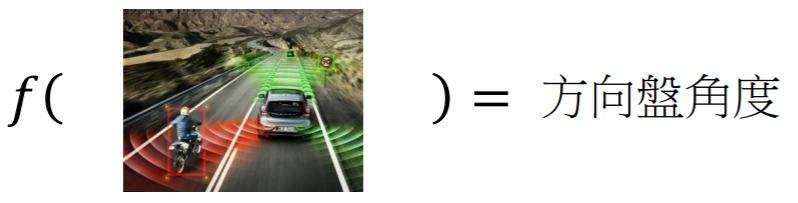• 推荐系统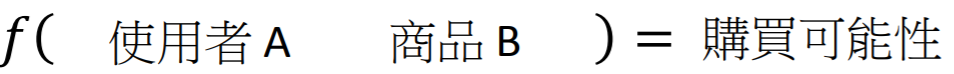## Regression应用举例

Input：Pokemon精灵的种种特性 $x$（例如：当前Pokemon精灵进化前的CP值 $x_{cp}$、物种 $x_s$、HP值 $x_{hp}$、重量 $x_w$、高度 $x_h$…）

Output：Pokemon精灵进化后的攻击力（CP值）

### Step 1：Model—Linear Model（线性模型）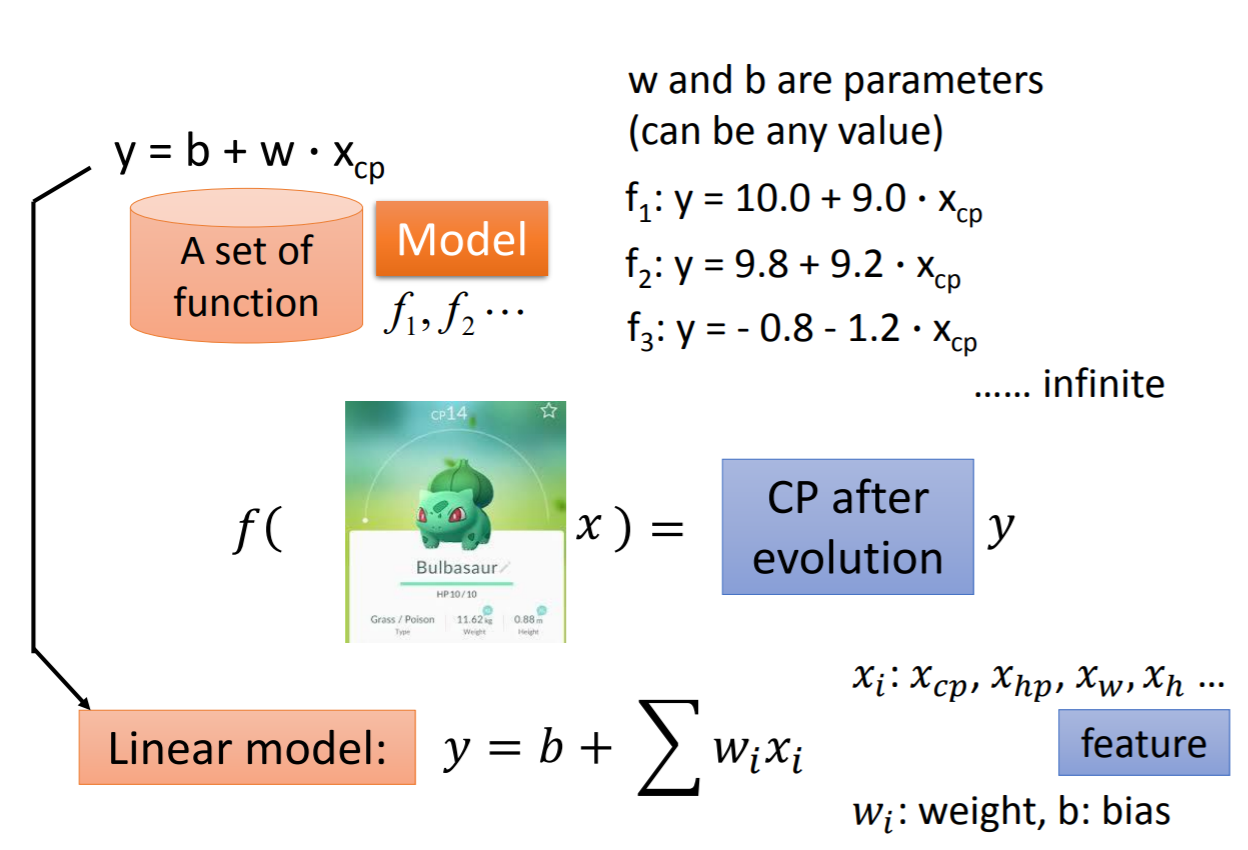$y = b+\sum\omega_ix_i$

### Step 2：Goodness of Function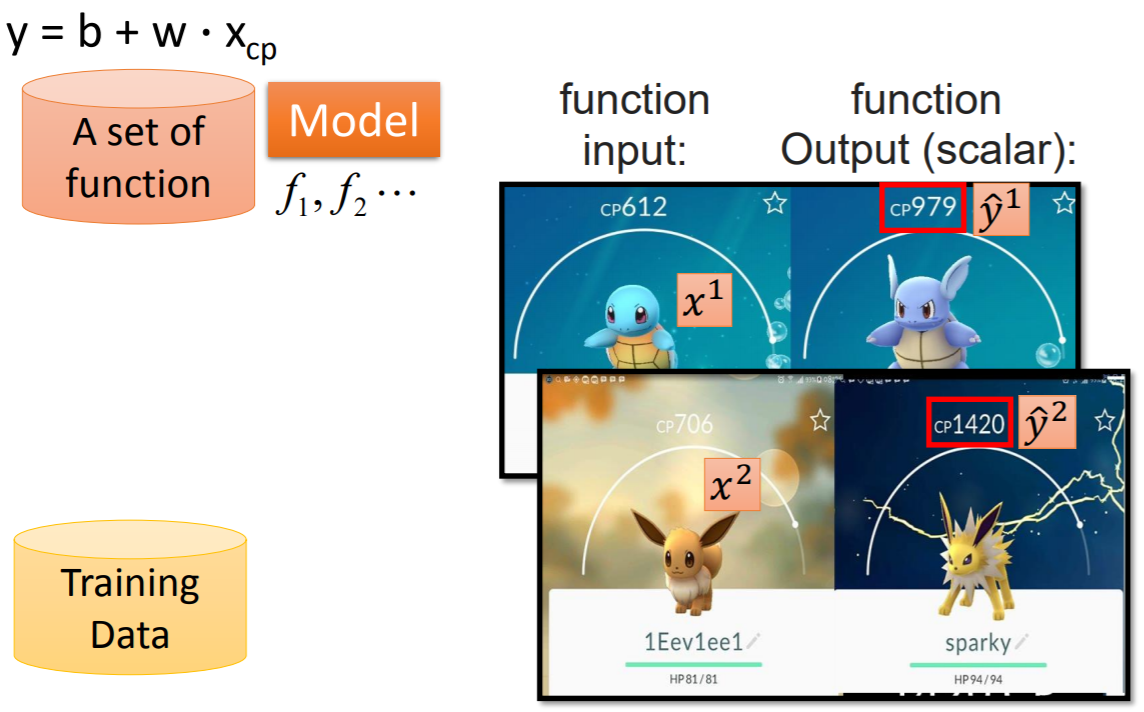$((x^1,\hat{y}^1),(x^2,\hat{y}^2),...,(x^{n-1},\hat{y}^{n-1}),(x^n,\hat{y}^n))$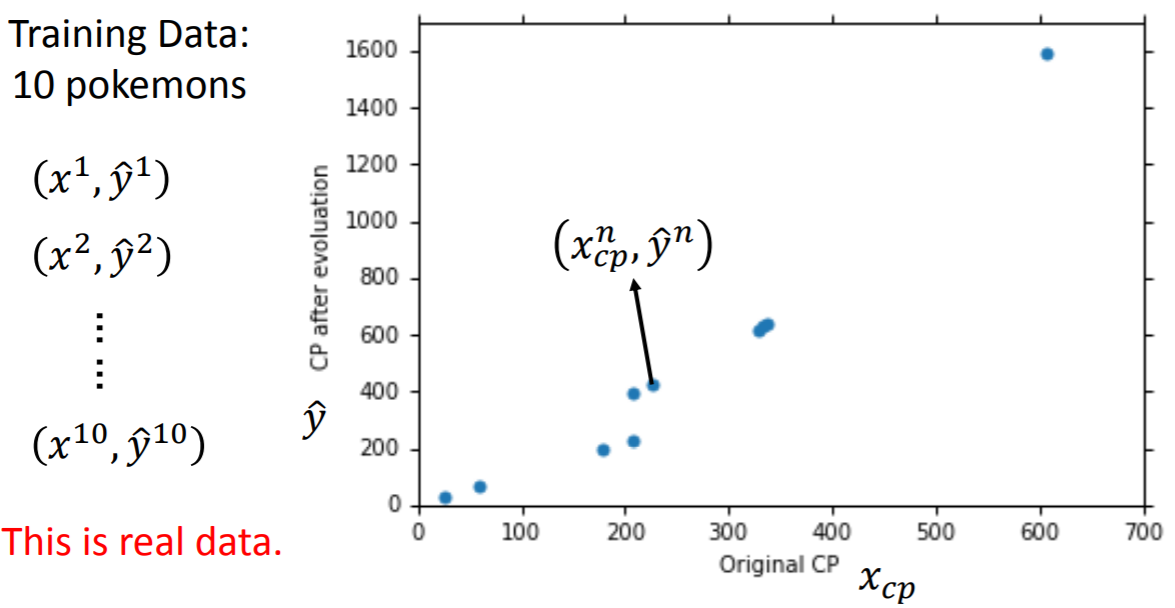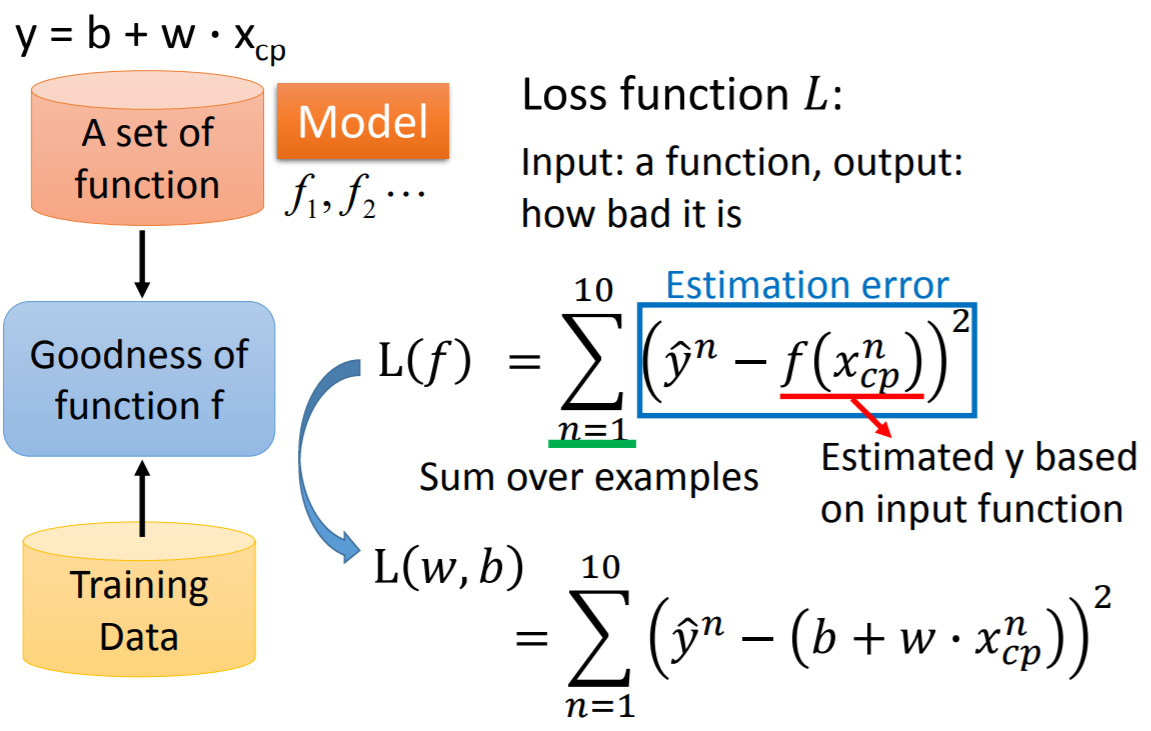$L(f) = \sum_{n=1}^{10}(\hat{y}^n-f(x_{cp}^n))^2$

$L(\omega,b) = \sum_{n=1}^{10}(\hat{y}^n - (b+\omega \cdot x_{cp}^n))^2$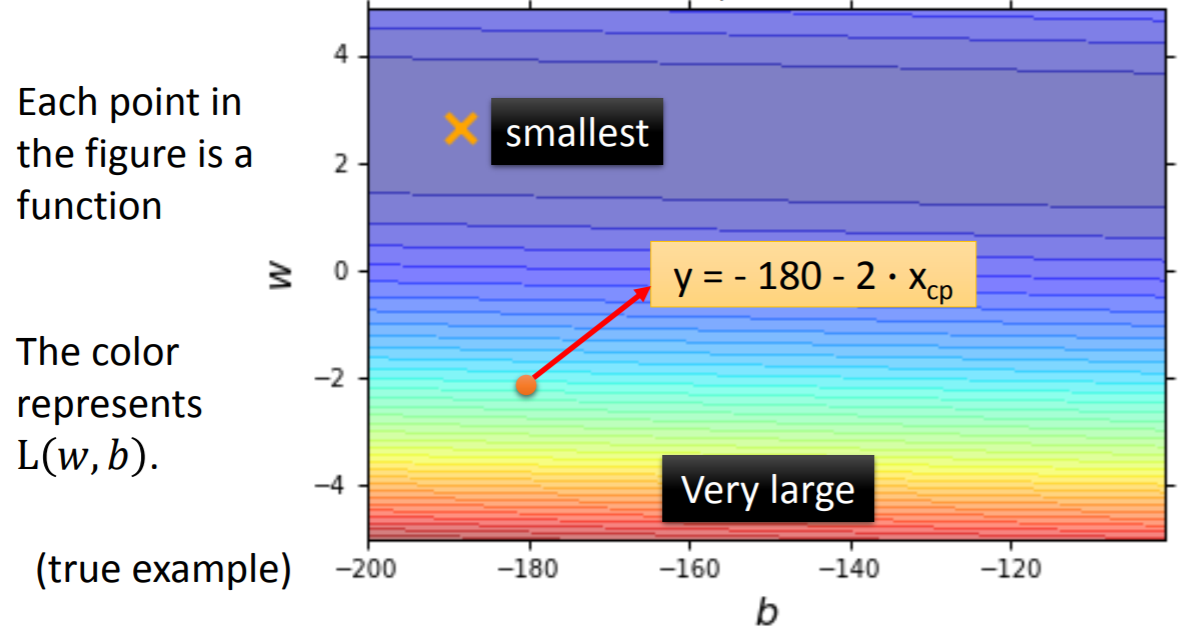### Step3：Best Function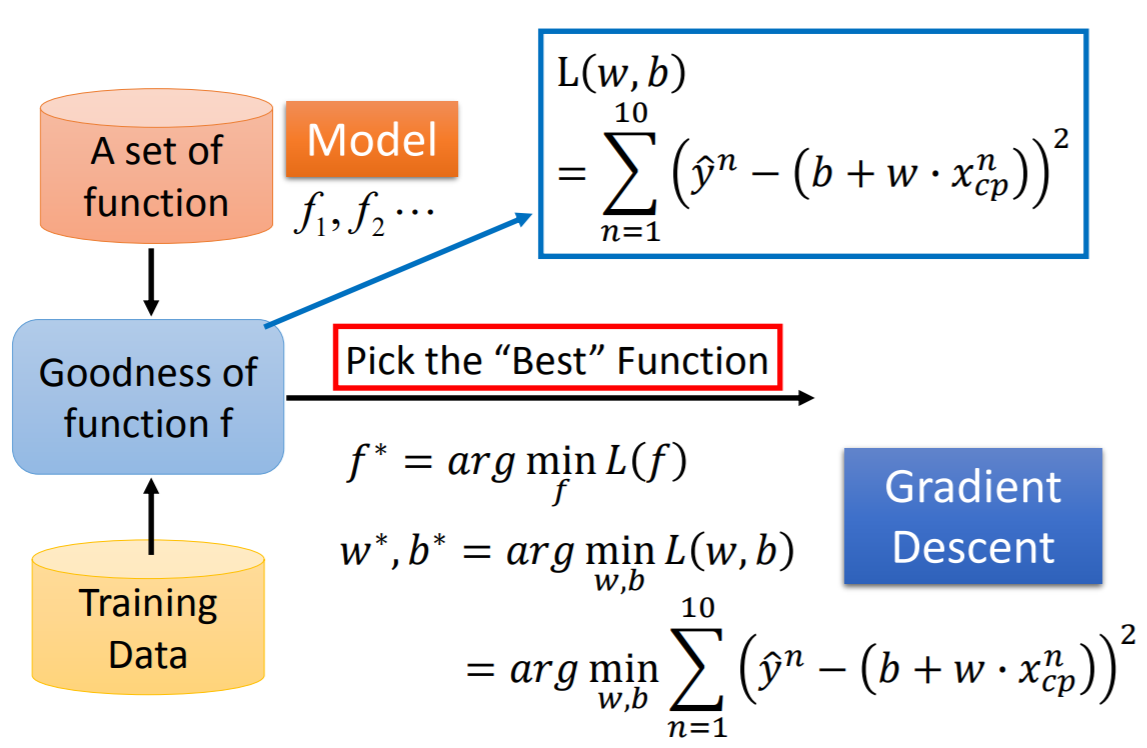$f^* = arg \min_f L(f)$

$\omega^*,b^* = arg \min_{\omega,b} L(\omega,b) = arg\min_{\omega,b}\sum_{n=1}^{10}(\hat{y}^n - (b+\omega \cdot x_{cp}^n))^2$

$\omega^* = arg \min_{\omega} L(\omega)$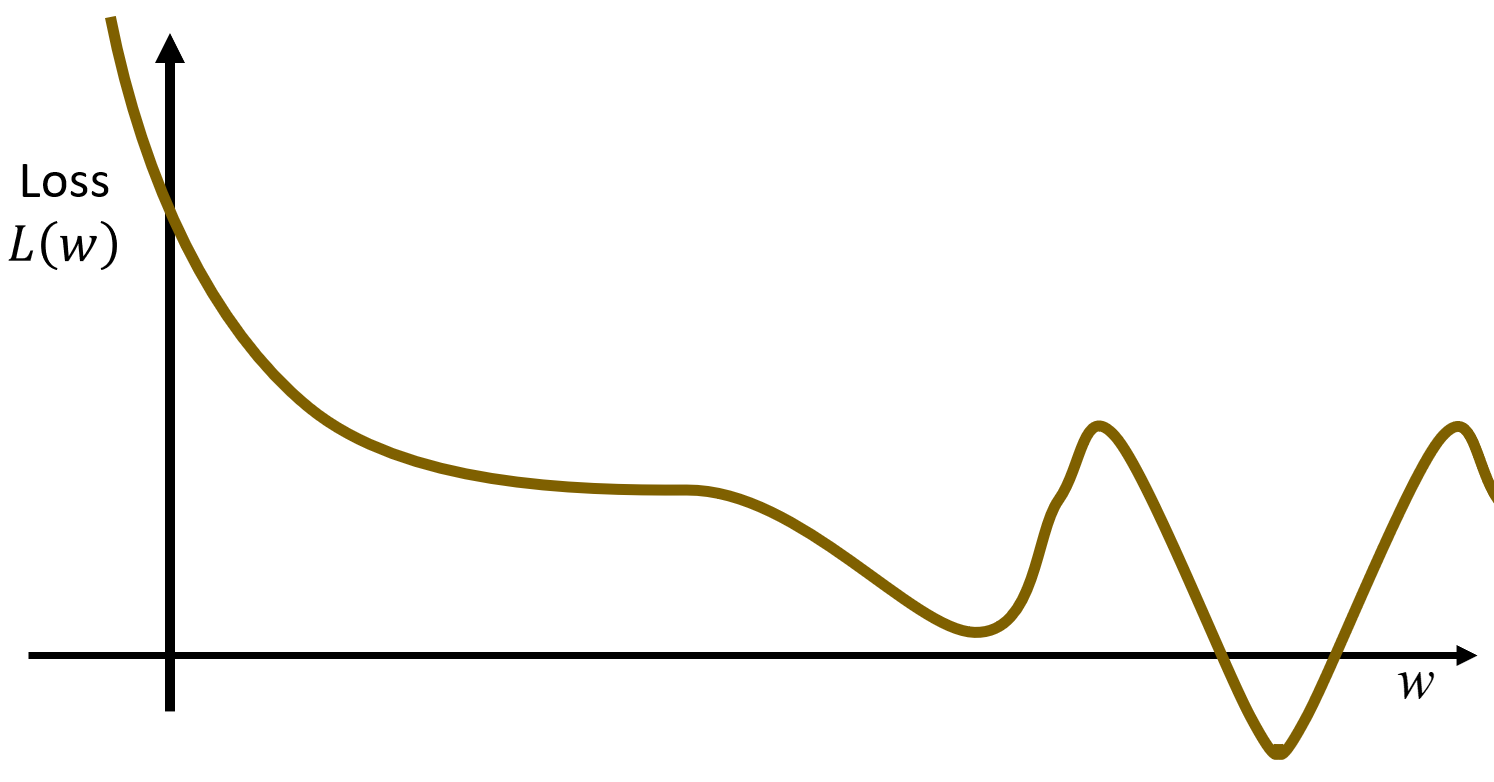• 随机初始化 $\omega^0$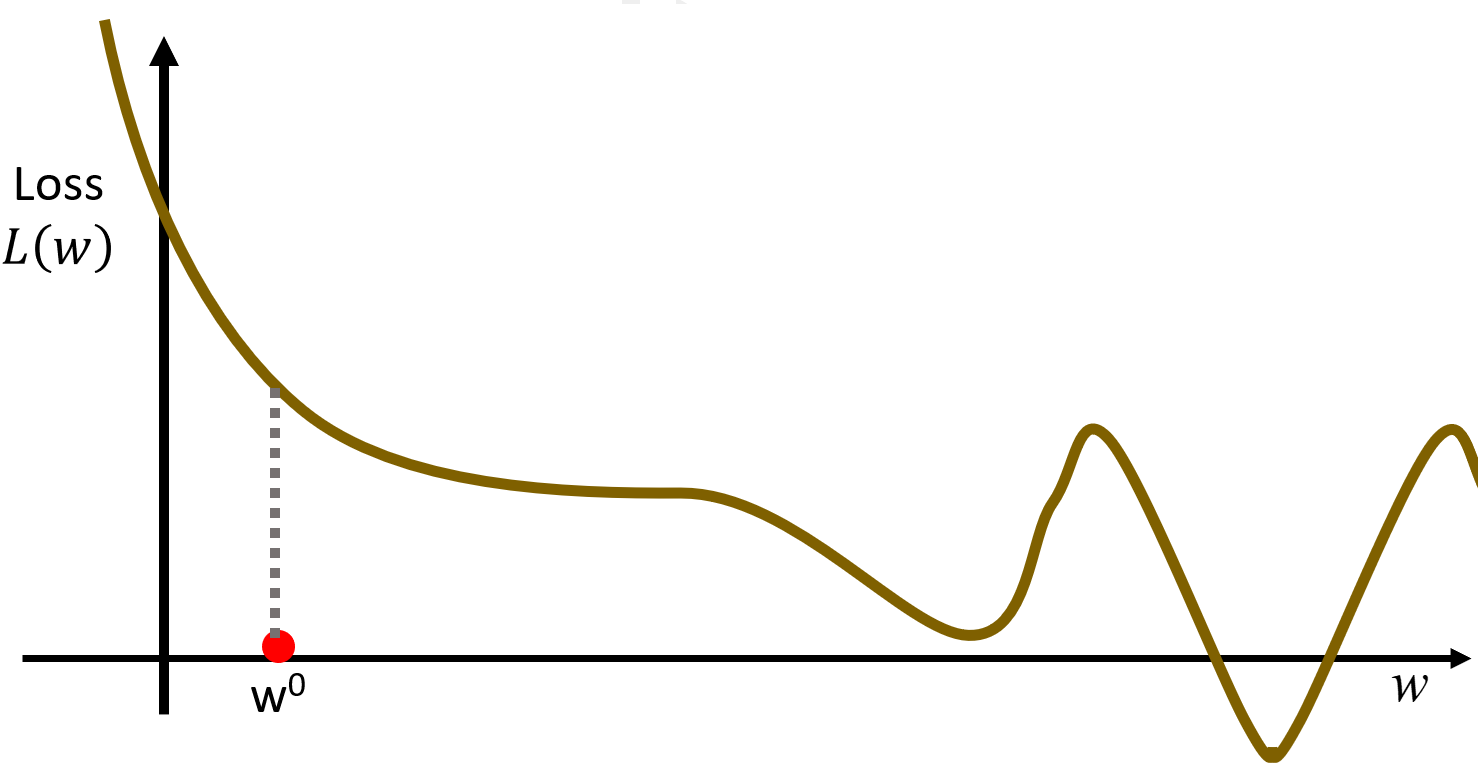• 计算微分 $\frac{dL}{d\omega}|_{\omega = \omega^0}$（在二维空间上，微分即为函数对应点的导数）。假设导数是负的，则意味着函数在递减，这是需要将参数 $\omega$向右移动，使得 $L(\omega)$变小；反之，假设导数是正的，则意味着函数在递曾，这是需要将参数 $\omega$向左移动，使得 $L(\omega)$变小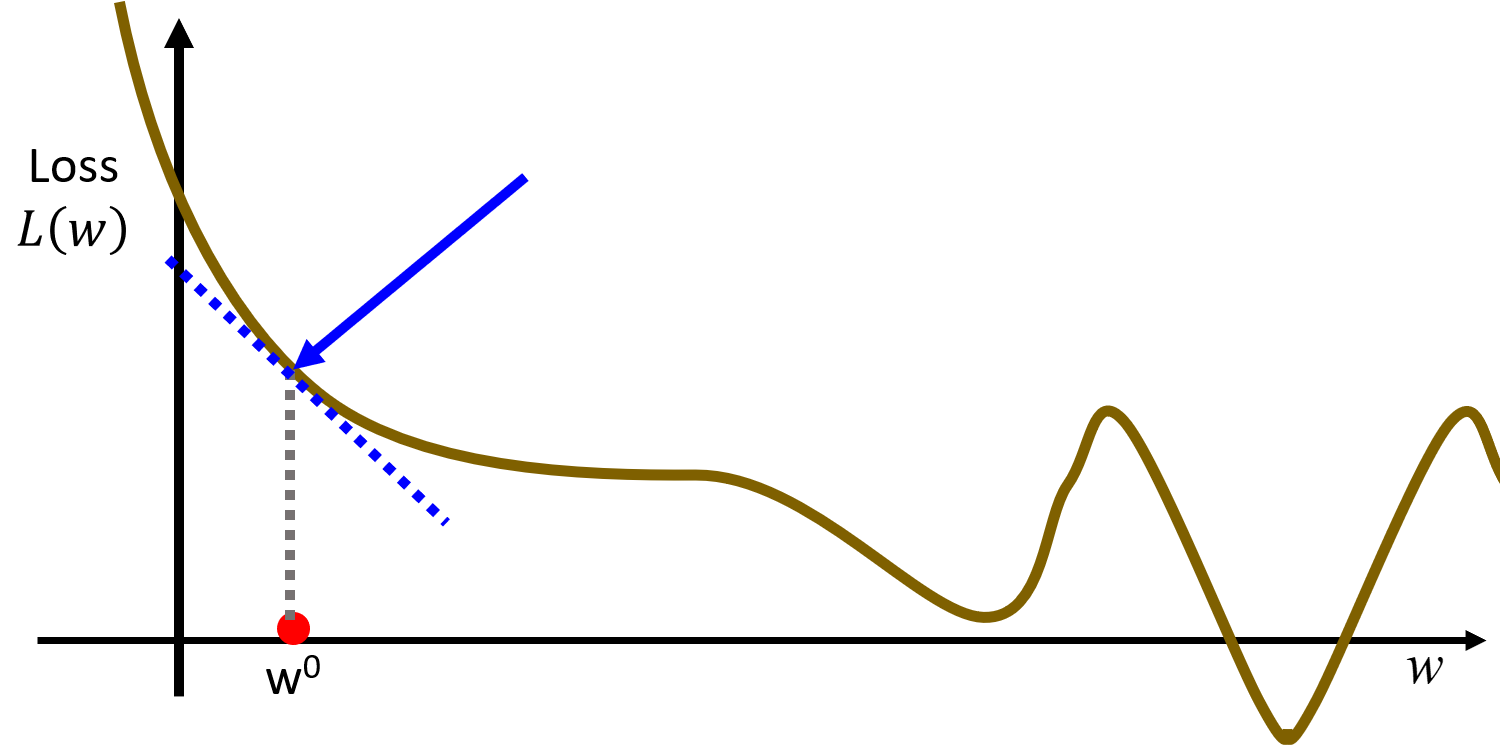• 更新 $\omega$
$\omega^1\leftarrow\omega^0 - \eta \frac{dL}{d\omega}|_{\omega = \omega^0}$
其中， $\eta$代表learning rate(学习率)，它决定了你学习的速度有多快。 $\eta$不能太大也不能太小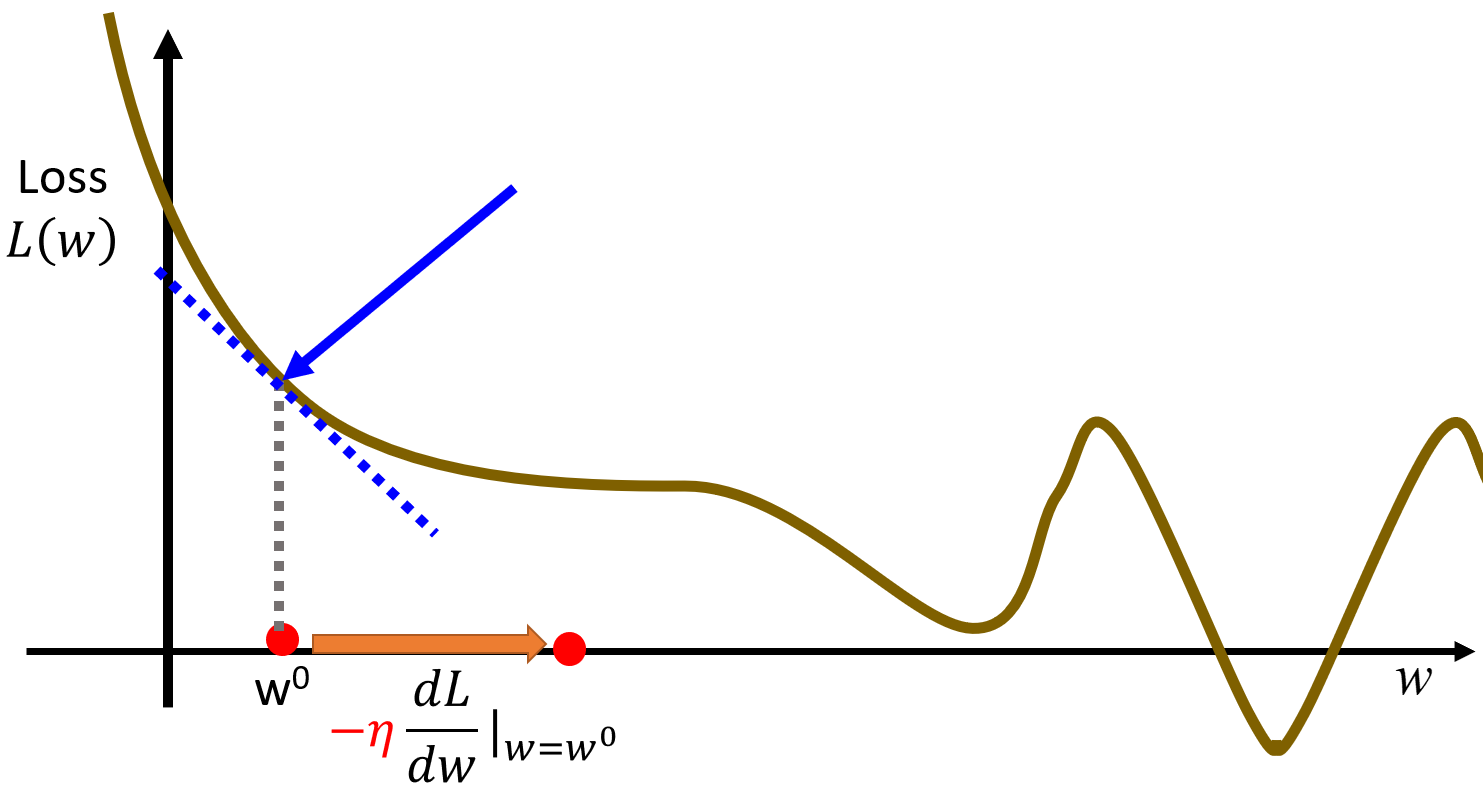• 重新开始第2步，计算微分 $\frac{dL}{d\omega}|_{\omega = \omega^1}$，直到计算出来的微分为0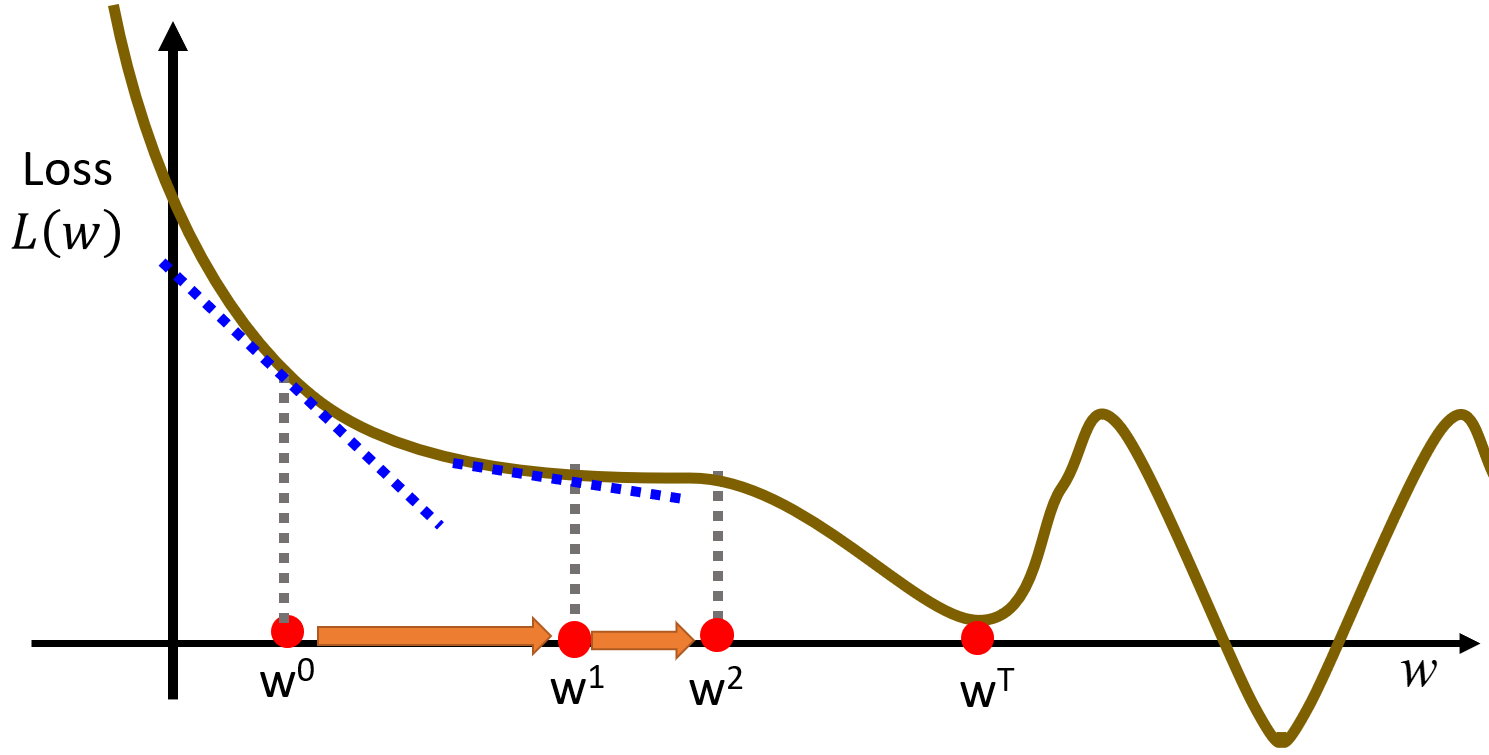$\omega^*,b^* = arg \min_{\omega,b} L(\omega,b)$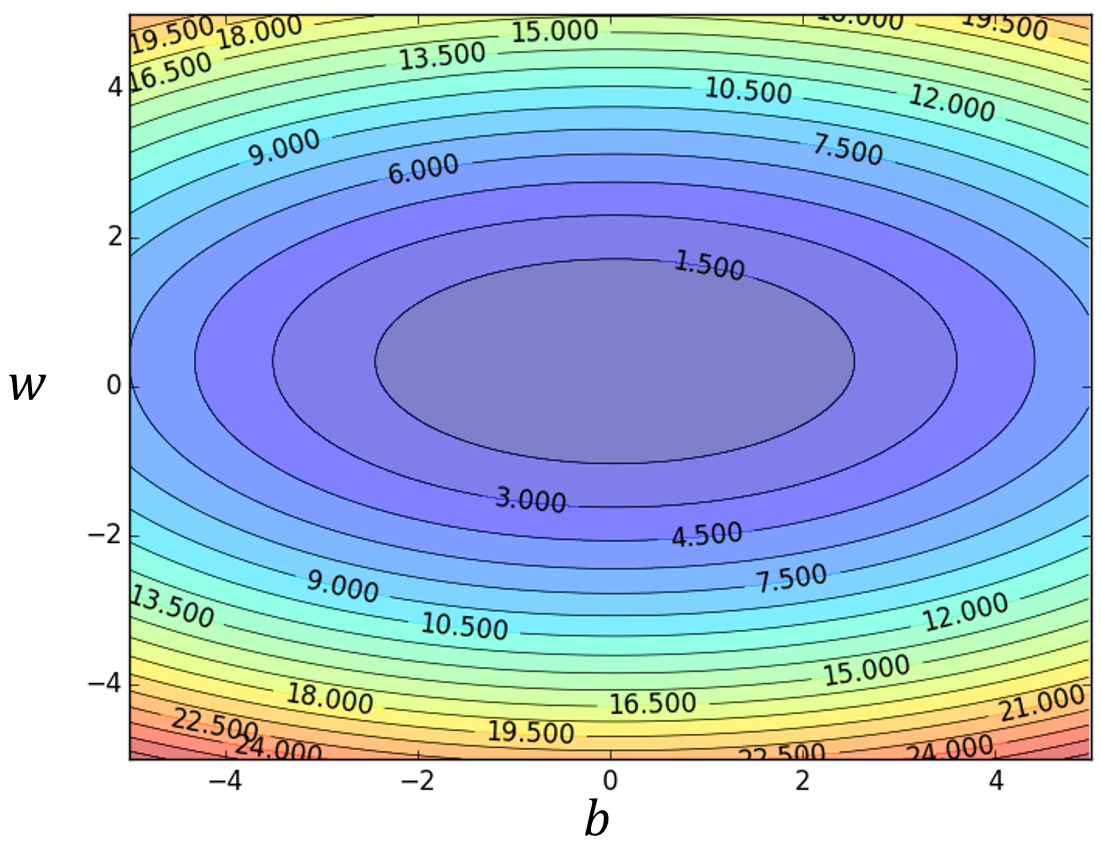• 随机初始化 $\omega$ $b$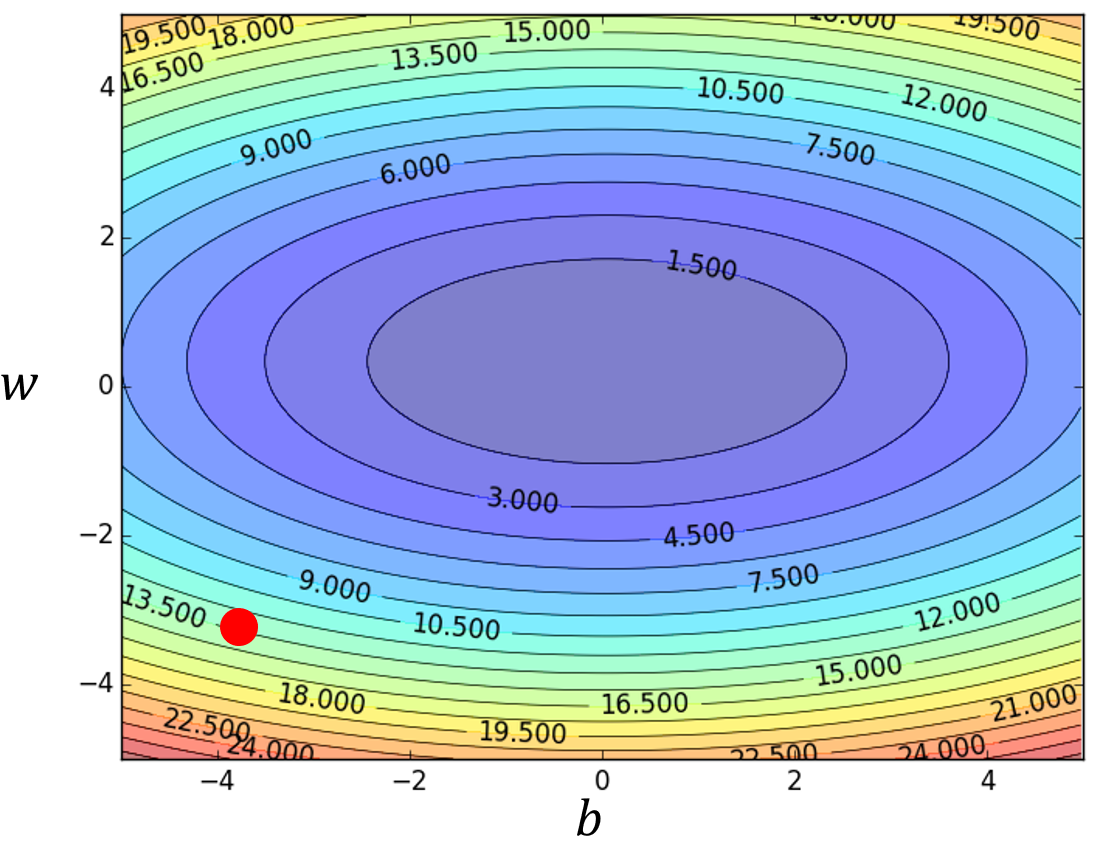• 分别计算微分 $\frac{\partial{L}}{\partial{\omega}}|_{\omega = \omega^0,b = b^0},\frac{\partial{L}}{\partial{b}}|_{\omega = \omega^0,b = b^0}$
$L(\omega,b) = \sum_{n=1}^{10}(\hat{y}^n - (b+\omega \cdot x_{cp}^n))^2$
$\frac{\partial{L}}{\partial{\omega}} = \sum_{n=1}^{10}2(\hat{y}^n - (b+\omega \cdot x_{cp}^n))(-x_{cp}^n)$
$\frac{\partial{L}}{\partial{b}} = \sum_{n=1}^{10}2(\hat{y}^n - (b+\omega \cdot x_{cp}^n))(-1)$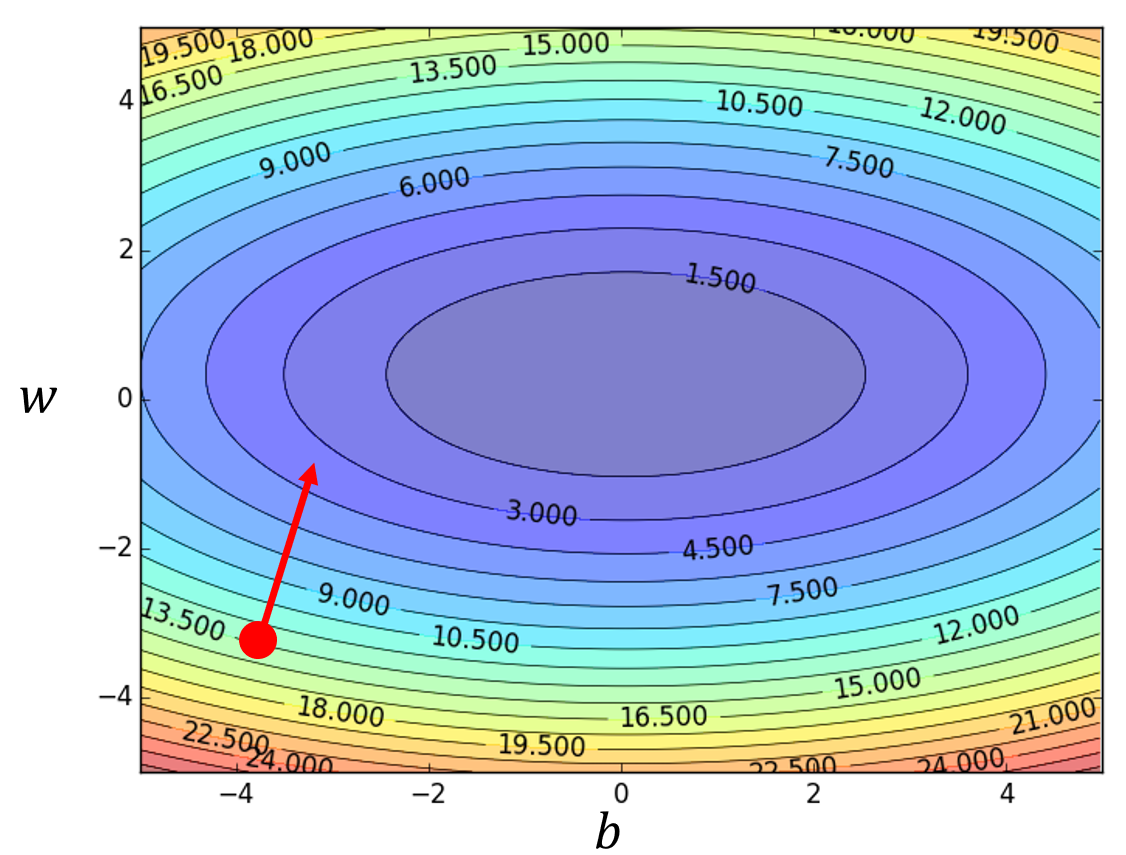• 更新 $\omega$ $b$
$\omega^1\leftarrow\omega^0 - \eta \frac{\partial{L}}{\partial{\omega}}|_{\omega = \omega^0,b = b^0} \ \ \ \ \ \ \ \ \ \ \ \ b^1\leftarrow b^0 - \eta \frac{\partial{L}}{\partial{b}}|_{\omega = \omega^0,b = b^0}$
而由 $\frac{\partial{L}}{\partial{\omega}}$ $\frac{\partial{L}}{\partial{b}}$构成Gradient(梯度)为：

$\nabla L = \left[\begin{matrix} \frac{\partial{L}}{\partial{\omega}} \\ \frac{\partial{L}}{\partial{b}} \end{matrix}\right]_{gradient}$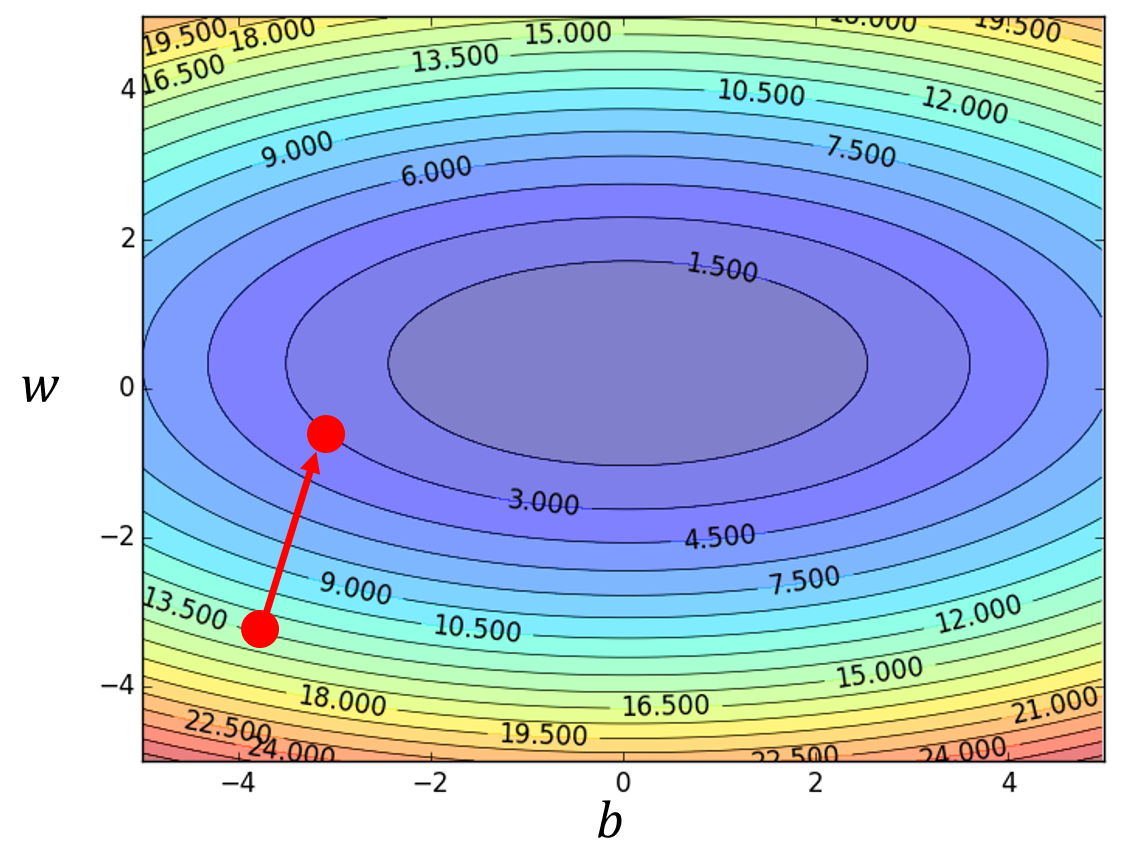• 重新开始第2步，计算微分 $\frac{\partial{L}}{\partial{\omega}}|_{\omega = \omega^1,b = b^1},\frac{\partial{L}}{\partial{b}}|_{\omega = \omega^1,b = b^1}$，直到计算出来的微分均为0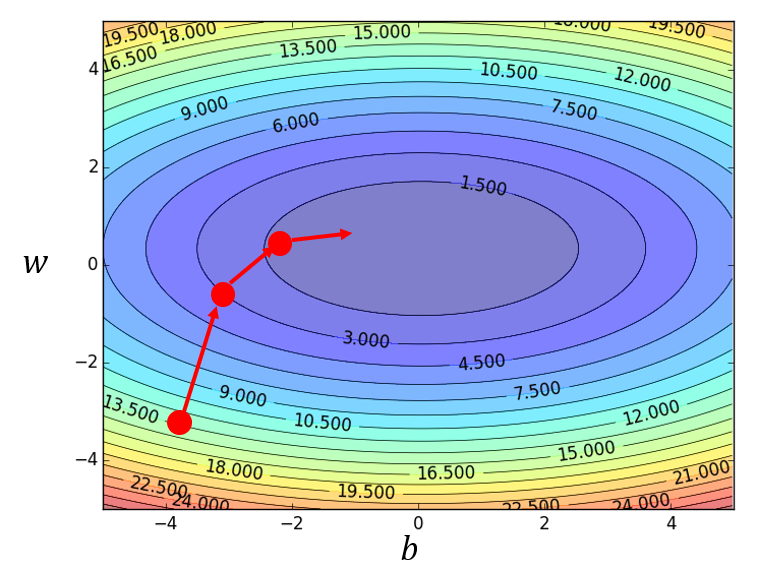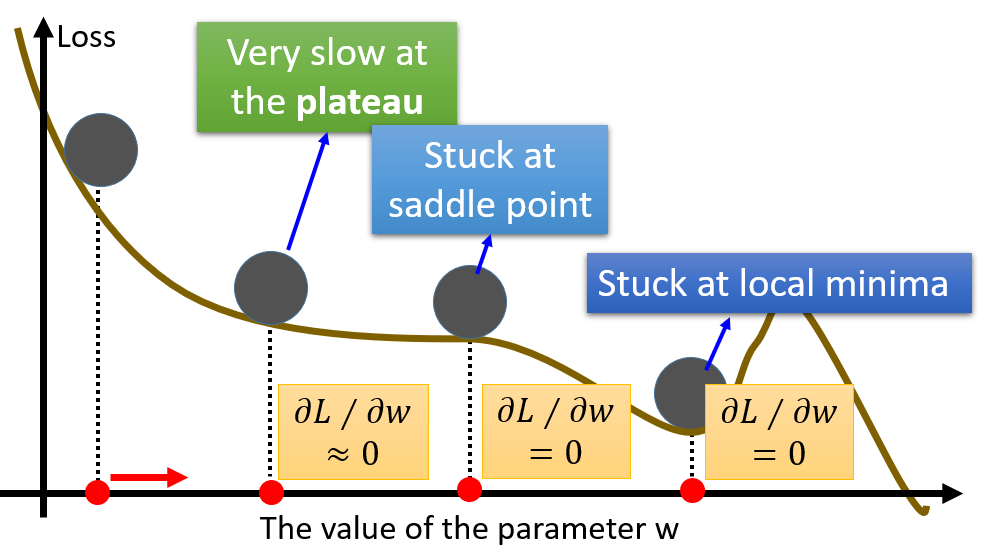• Stuck at the Local Minima（局部最小）

• Stuck at the Saddle Point（鞍点）

• Very slow at the Plateau(一些特殊的地形，如大面积的平坦区域）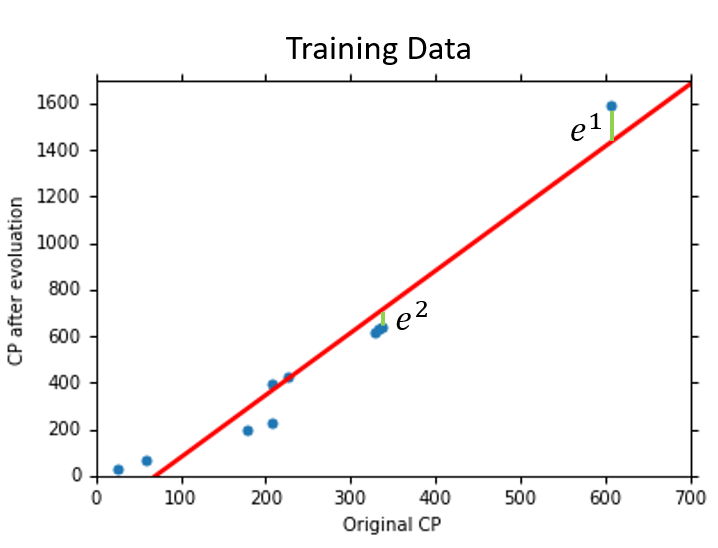$b = -10.3\ \ \ \omega_1 = 1.0\ \ \ \omega_2 = 2.7\times 10^{-3}\ \ \ Error_{train} = 15.4\ \ \ Error_{test} = 18.4$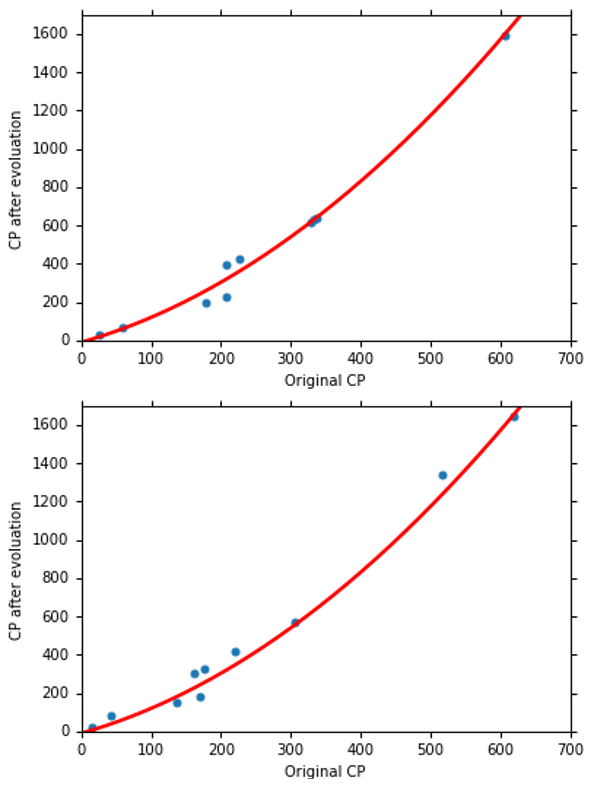$b = 6.4\ \ \ \omega_1 = 0.66\ \ \ \omega_2 = 4.1\times 10^{-3}\ \ \ \omega_3 = -1.8\times 10^{-6}\ \ \ Error_{train} = 15.3\ \ \ Error_{test} = 18.1$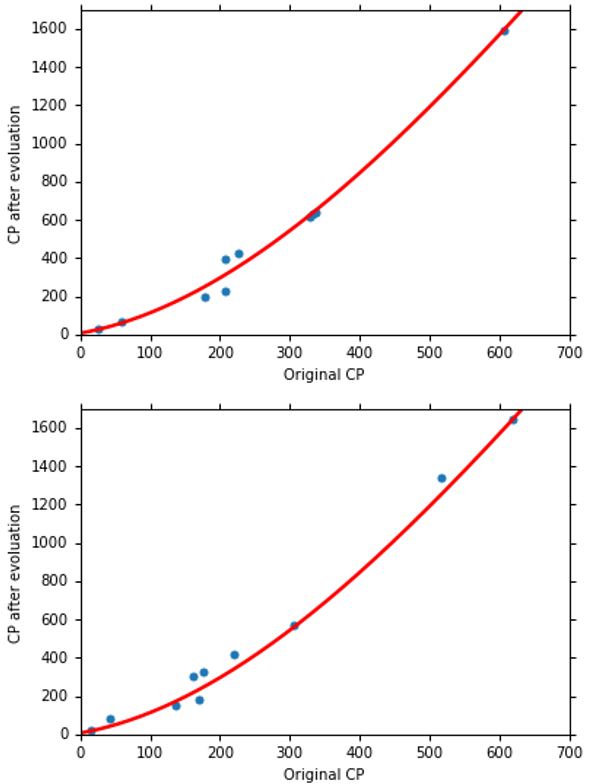$Error_{train} = 14.9\ \ \ Error_{test} = 28.8$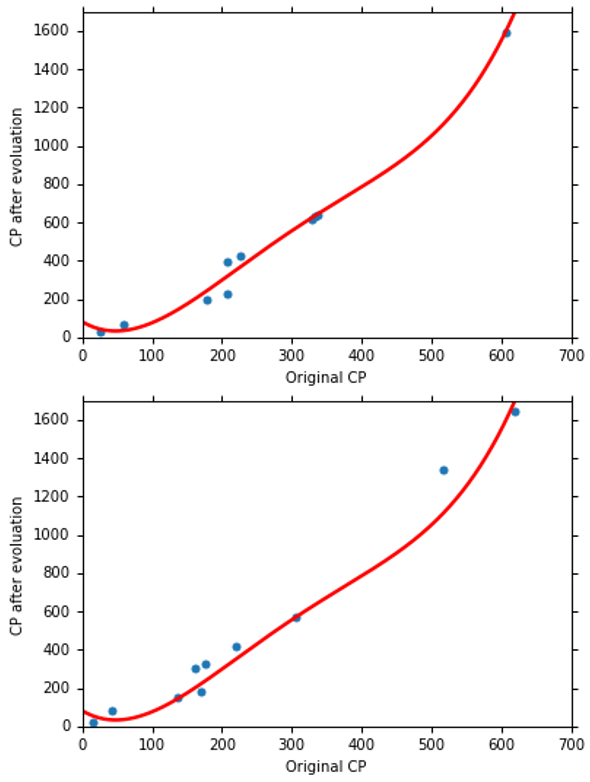$Error_{train} = 12.8\ \ \ Error_{test} = 232.1$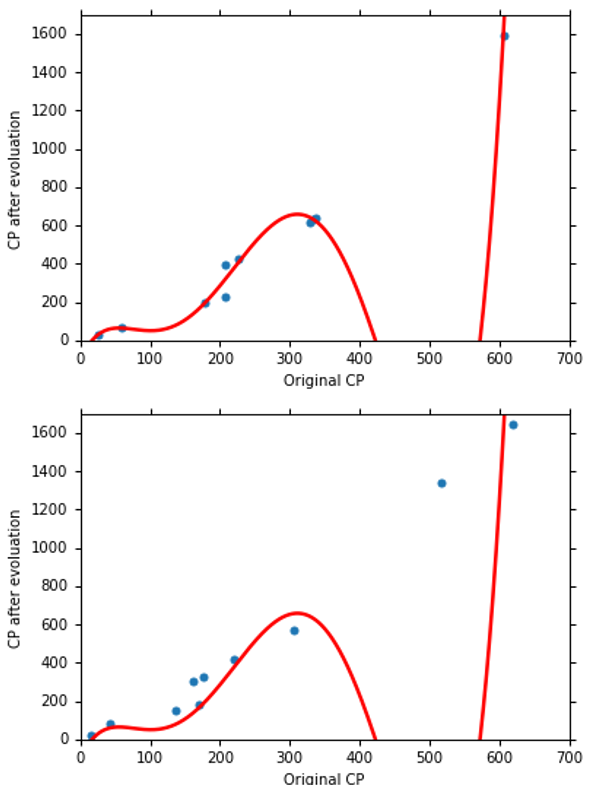• 对于Training Data（训练数据）：
我们知道，对于每个Model来说其实都是一个Function Set，当Model越复杂时，Function Set越大且包含了简单Model的Function Set，比如三次Model的Function Set其实是四次Model的Function Set（ $\omega_4 = 0$）的一个子集合。所以，当Model越复杂时，我们能在更多的Function中挑选最好的Function，从而使得Training Data的Error越低。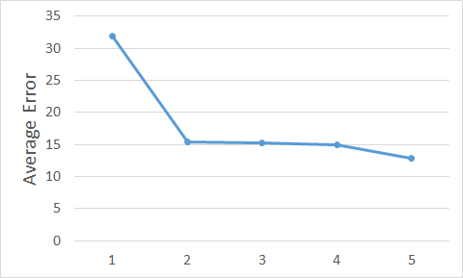• 对于Testing Data（测试数据）：
我们发现，三次Model的Performance（效果）是最好的。当Model越复杂时，Error就会突然爆炸，所以一个复杂的Model，并不一定总会在Testing Data（测试数据）给我们一个好的Performance。这是Overfitting（过拟合）现象。Overfitting（过拟合）的根本原因是特征维度过多，模型假设过于复杂，参数过多，训练数据过少，噪声过多，导致拟合的函数完美的预测训练集，但对新数据的测试集预测结果差。过度的拟合了训练数据，而没有考虑到Generalization（泛化）能力。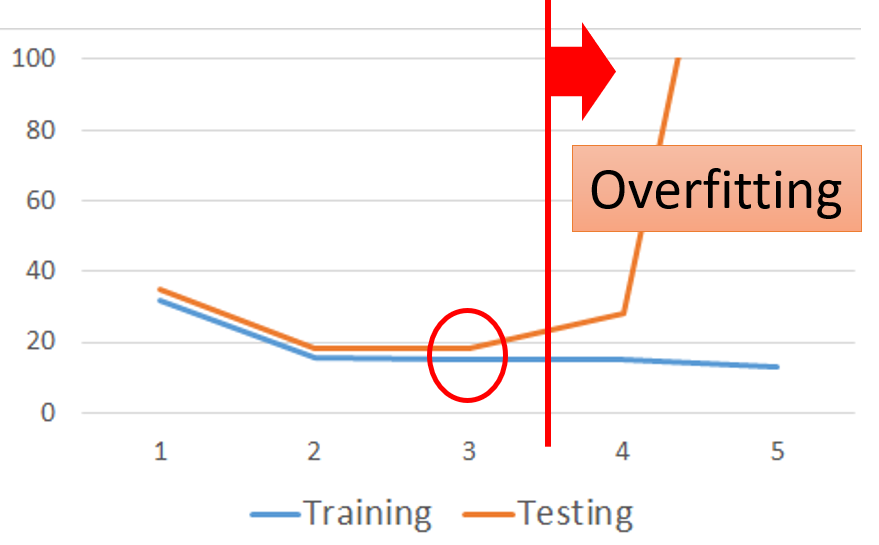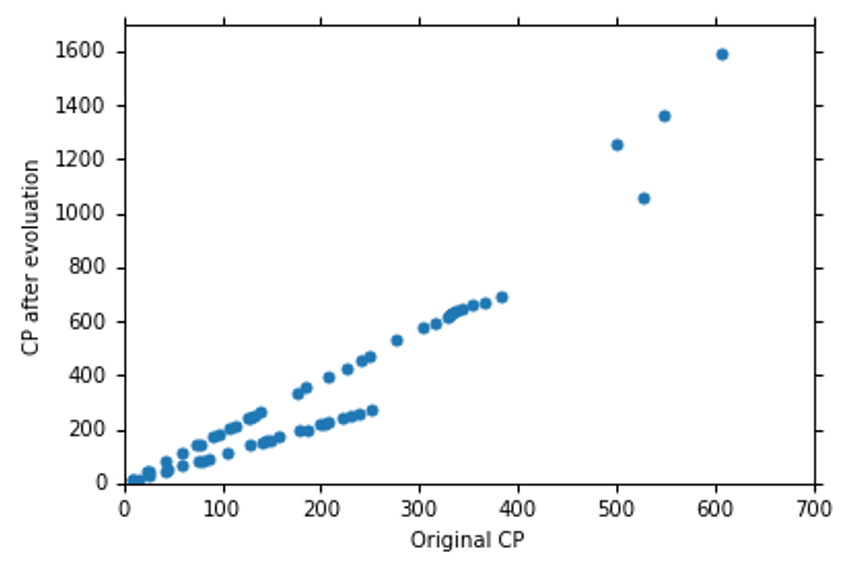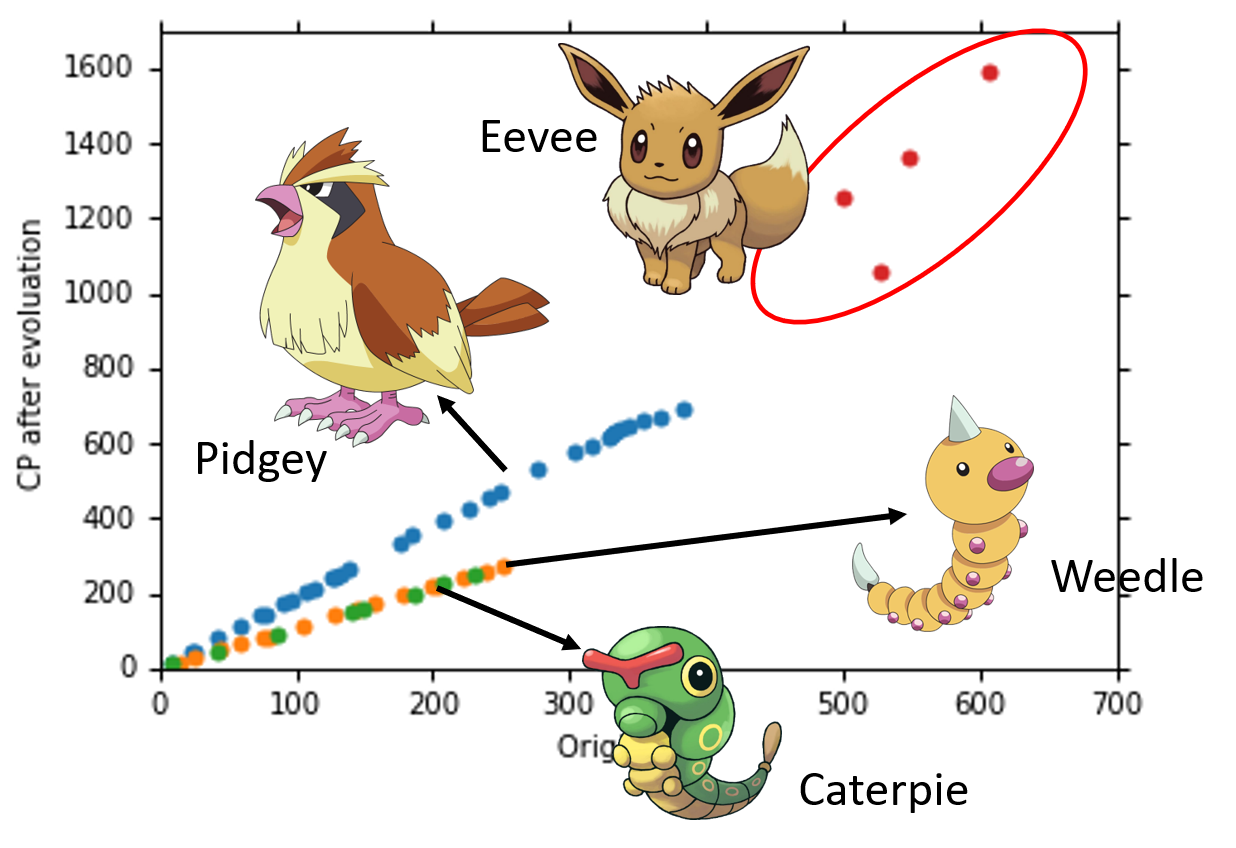### Back to Step 1：Redesign the Model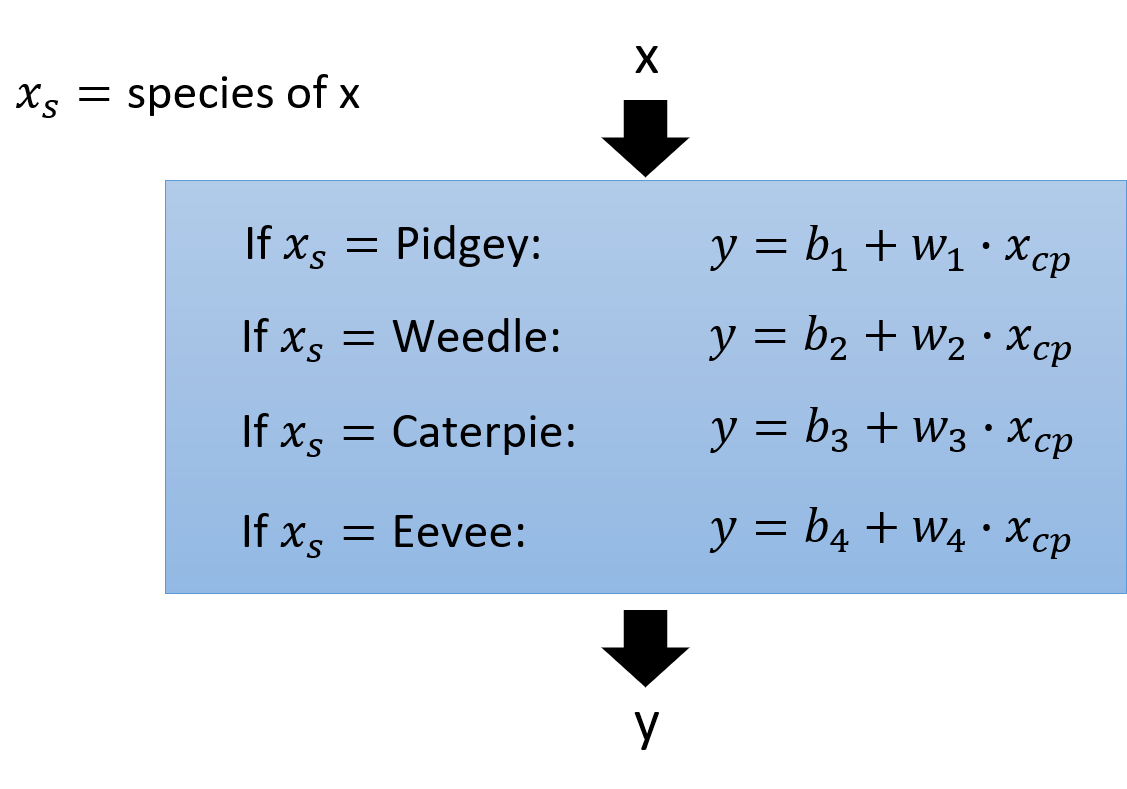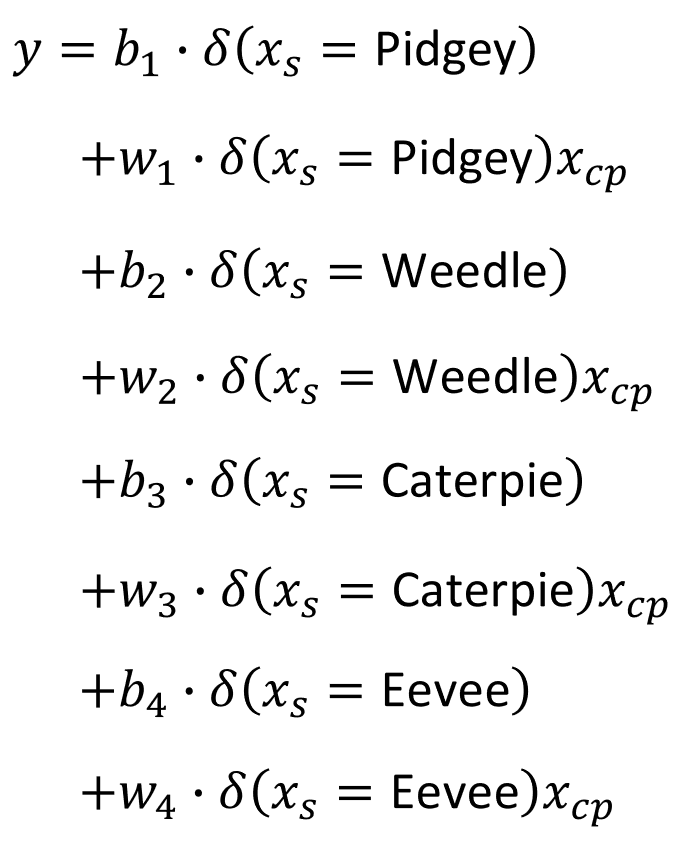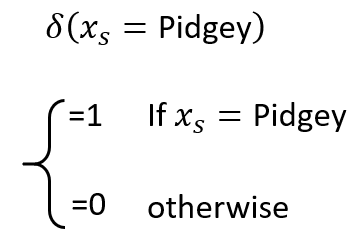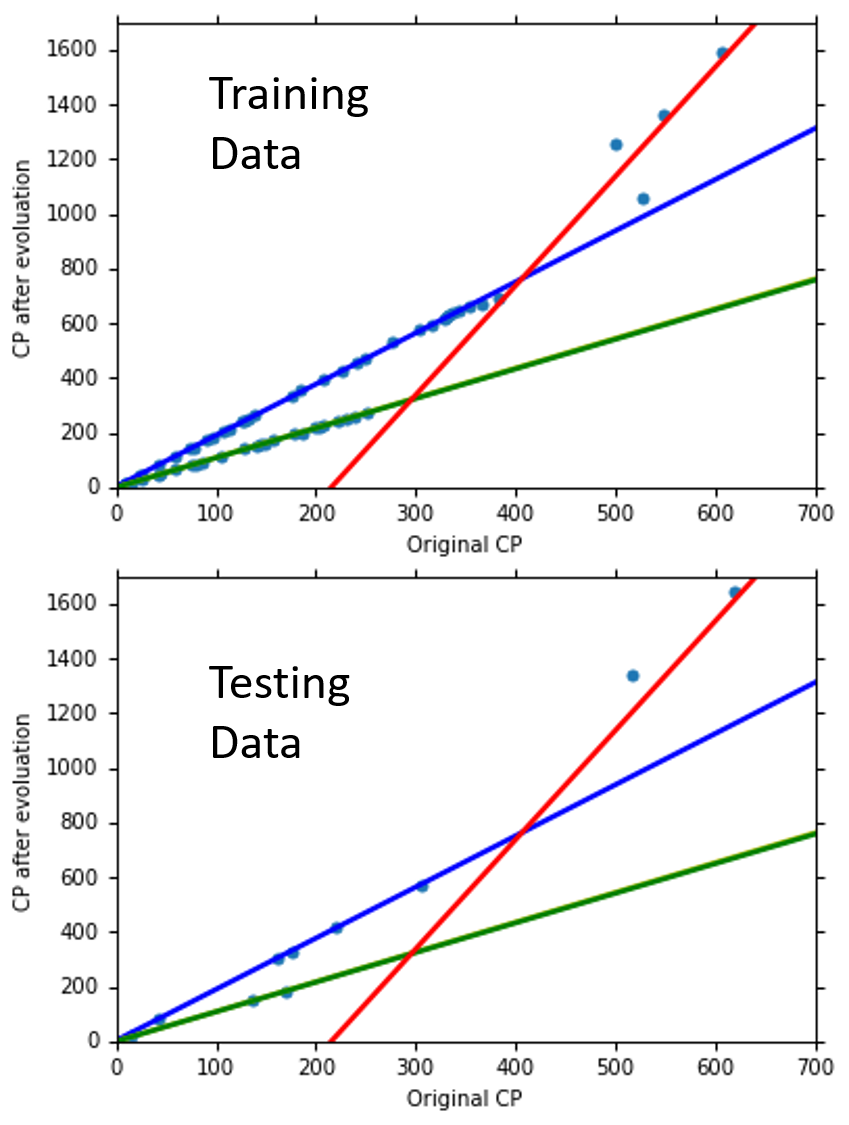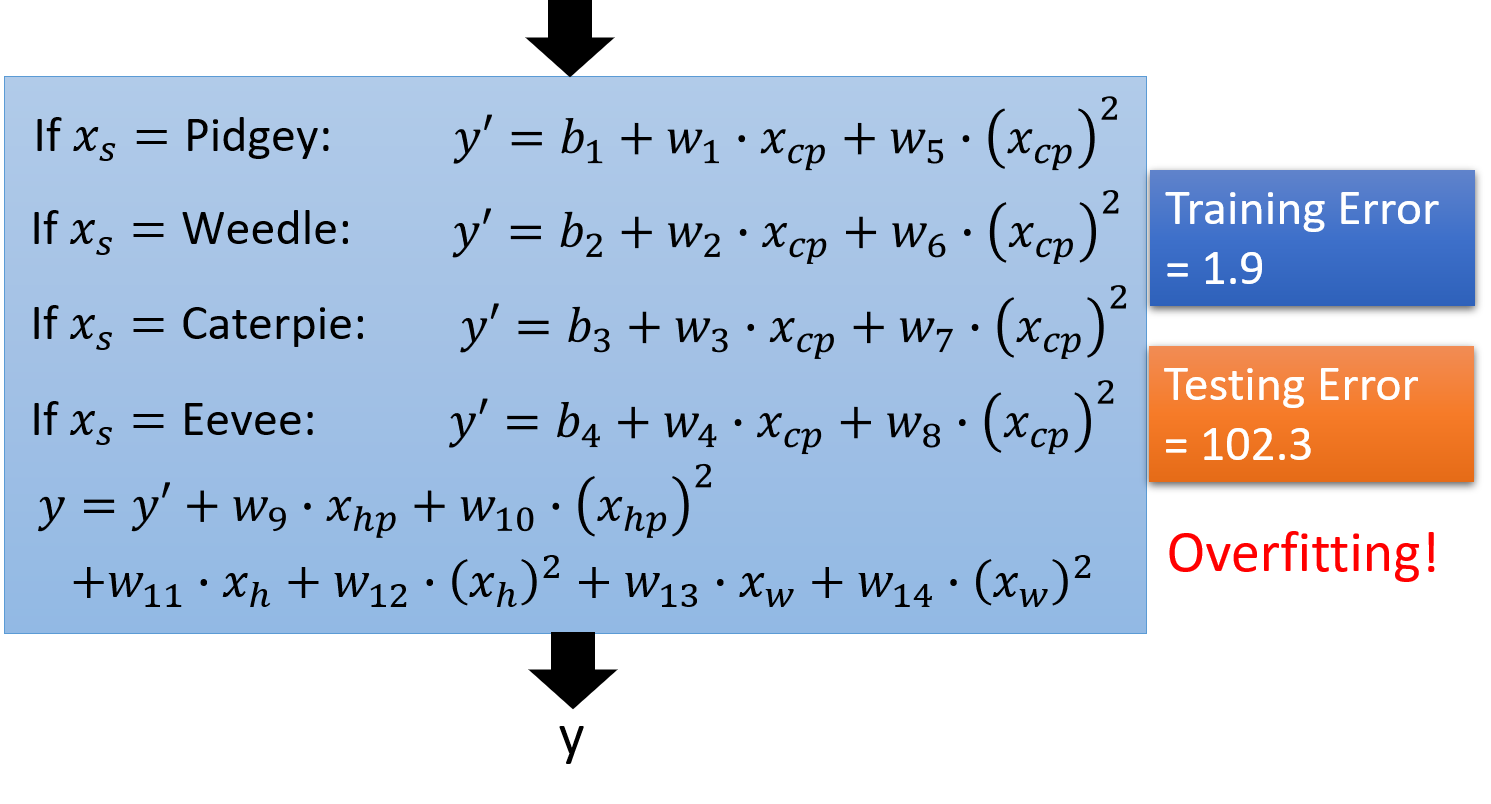$L(\omega,b) = \sum_{n=1}^{10}(\hat{y}^n - (b+\omega \cdot x_{cp}^n))^2$

$L(\omega,b) = \sum_{n=1}^{10}(\hat{y}^n - (b+\omega \cdot x_{cp}^n))^2 + \lambda\sum(\omega_i)^2$

$\omega$很小的Function意味着它是一个比较smooth（平滑）的Function。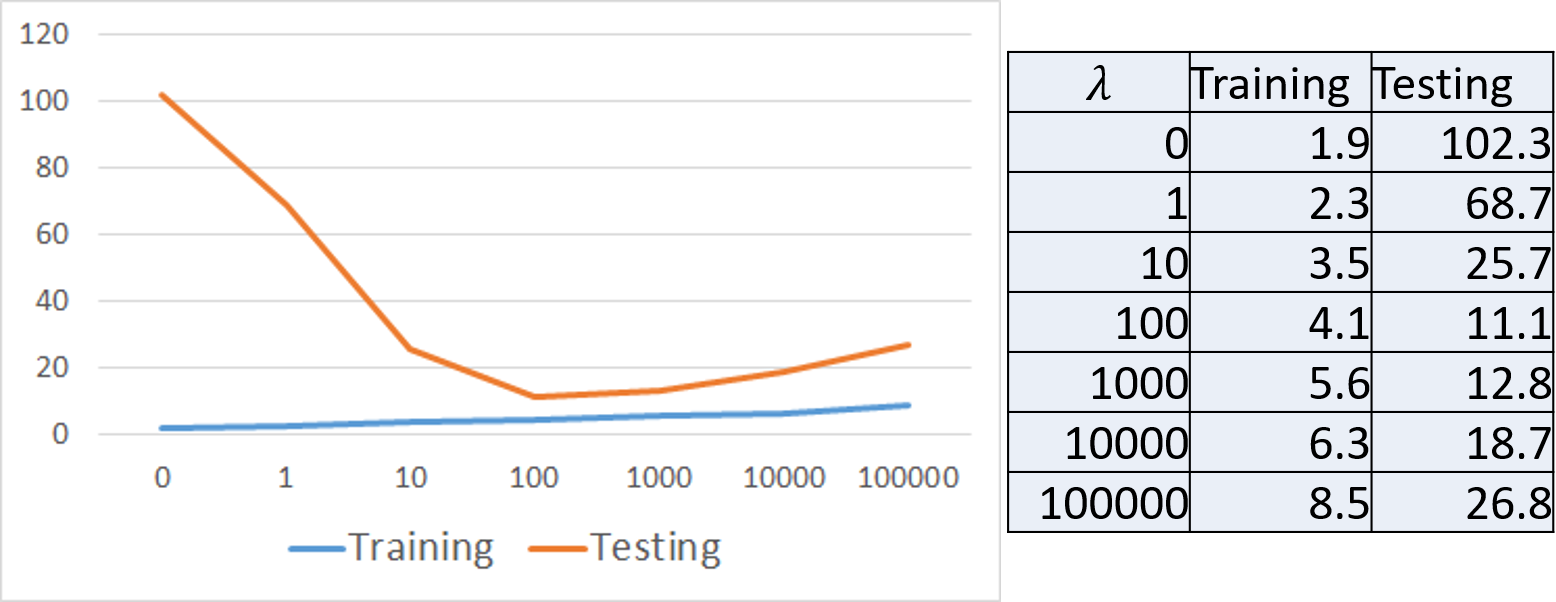• 对于 $Error_{train}$, $\lambda$越大， $Error_{train}$越大
• $\lambda$并不是越大越好，需要选择一个最合适的 $\lambda$，才能得到最好的结果

## Conclusion（结论）

• Pokemon精灵进化后的CP值和Pokemon的物种以及进化前的CP值有关系，不可否认也可能有其他的因素影响了Pokemon精灵进化后的CP值
• 最终的Testing Data的Error为11.1

## Demo

import numpy as np
import matplotlib.pyplot as plt

x_data = [338.,333.,328.,207.,226.,25.,170.,60.,208.,606.]
y_data = [640.,633.,619.,393.,428.,27.,193.,66.,226.,1591.]
# ydata = b + w * xdata
x = np.arange(-200, -100, 1)
y = np.arange(-5, 5, 0.1)
X, Y = np.meshgrid(x, y)
z = np.zeros((len(x), len(y)))

for i in range(len(x)):
for j in range(len(y)):
b = x[i]
w = y[j]
z[j][i] = 0
for n in range(len(x_data)):
z[j][i] = z[j][i] + (y_data[n] - b - w*x_data[n])**2
z[j][i] = z[j][i] / len(x_data)

b = -120 # initial b
w = -4   # initial w
lr = 1 # learning rate
iteration = 1000000
# store initial value for plotting
b_history = [b]
w_history = [w]

lr_b = 0
lr_w = 0

# iteration
for i in range(iteration):

for n in range(len(x_data)):

lr_b = lr_b + b_grad ** 2
lr_w = lr_w + w_grad ** 2

# update parameters
b = b - lr/np.sqrt(lr_b) * b_grad
w = w - lr/np.sqrt(lr_w) * w_grad

# b = b - lr*b_grad
# w = w - lr*w_grad

# store parameters for plotting
b_history.append(b)
w_history.append(w)

# plot the figure
plt.contourf(x, y, z, 50, alpha=0.5, cmap=plt.get_cmap('jet'))
plt.plot([-188.4], [2.67], 'x', ms=12, markeredgewidth=3, color='orange')
plt.plot(b_history, w_history, 'o-', ms=3, lw=1.5, color='black')
plt.xlim(-200, -100)
plt.ylim(-5, 5)
plt.xlabel(r'$b$', fontsize=16)
plt.ylabel(r'$w$', fontsize=16)
plt.show()